﻿ 基于金属光栅结构的SPP模式的研究

# 基于金属光栅结构的SPP模式的研究The Research of SPP Mode Based on Metallic Grating Structure

Abstract: Metal nanostructures are based on the principle of localized surface plasmon resonance on optical wavelengths. It can be controlled by changing various parameters (size, material, shape) of the structure and the environments in which they are located. This paper presents a metallic grating structure. The spectral characteristics of the structure and under different parameters and sensitivity under different environments are analyzed, and the resonance wavelength can be tuned in the near infrared band.

1. 引言

2. 金属纳米棒与金属光栅结构几何参数的分析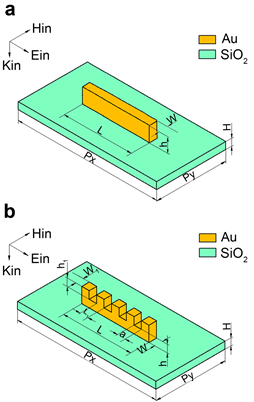Figure 1. Metal nanorods (a) and metal grating structures (b)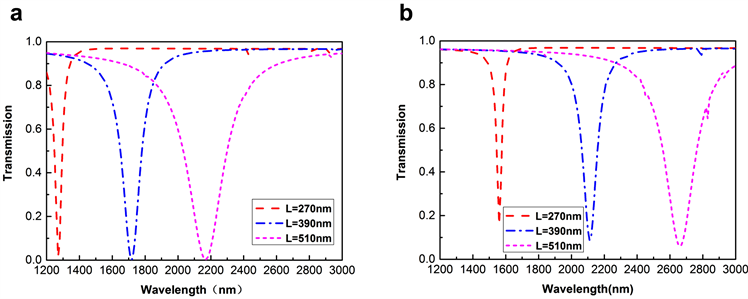Figure 2. Transmission of metal nanorods (a) and metal grating structures (b) when L values are different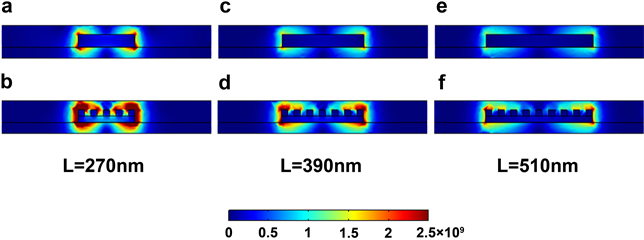Figure 3. Electric field strengths of metal nanorods (a, c, e) and metal grating structures (b, d, f) when L values are differentFigure 4. Charge distribution of metal nanorods (a) and metal grating structure (b) (L = 270 nm, N = 5)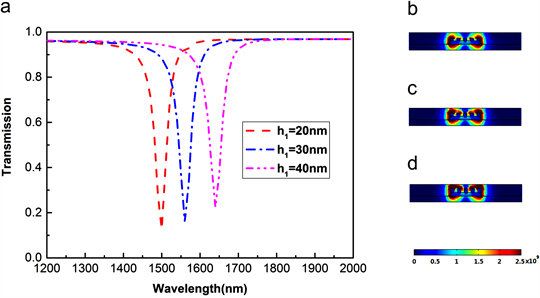Figure 5. Transmission (a) and corresponding electric field intensity of metal grating structure at different groove depths (b, h1 = 20 nm) (c, h1 = 30 nm) (d, h1 = 40 nm)

3. 金属纳米棒与金属光栅结构的灵敏度分析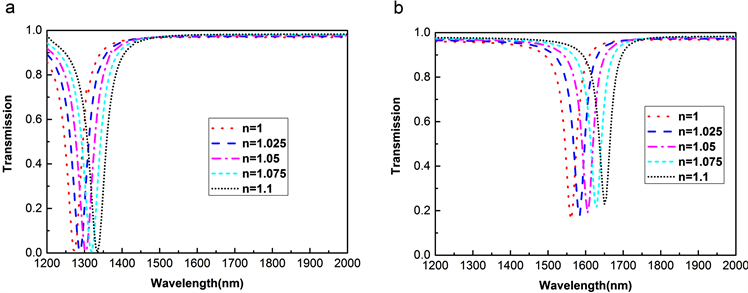Figure 6. Transmission of metal nanorods (a) and metal grating structures (b) in different environmentTable 1. Sensitivity Physical quantities of metal nanorods in different environmentTable 2. Sensitivity physical quantities of metal grating structures in different environment

${S}_{1}=\frac{\Delta \lambda }{\Delta n}=600\text{nm}/\text{RIU}$ (1)

${\text{FOM}}_{1}=\frac{{S}_{1}}{{\text{FWHM}}_{1}}=12.5$ (2)

${S}_{2}=\frac{\Delta \lambda }{\Delta n}=920\text{nm}/\text{RIU}$ (3)

${\text{FOM}}_{2}=\frac{{S}_{2}}{{\text{FWHM}}_{2}}=40$ (4)

4. 总结

NOTES

*通讯作者。

 Barnes, W.L., Dereux, A. and Ebbesen, T.W. (2003) Surface Plasmonsubwavelength Optics. Nature, 424, 824-830.
https://doi.org/10.1038/nature01937

 Ozbay, E. (2006) Plasmonics: Merging Photonics and Electronics Atnanoscale Dimensions. Science, 311, 189-193.
https://doi.org/10.1126/science.1114849

 Liu, H.T. and Lalanne, P. (2010) Light Scattering by Metallic Surfaces with Subwavelength Patterns. Physical Review B, 82, 115418.
https://doi.org/10.1103/PhysRevB.82.115418

 Hoeppener, S., Maoz, R., Cohen, S.R., Chi, L., Fuchs, H. and Sagiv, J. (2002) Metal Nanoparticles, Nanowires, and Contact Electrodes Self-Assembled on Patterned Monolayer Templates: A Bottom-Up Chemical Approach. Advanced Materials, 14, 5.

 Cubukcu, E., Kort, E.A., Crozier, K.B. and Capasso, F. (2006) Plasmonic Laser Antenna. Applied Physics Letters, 89, 093120.
https://doi.org/10.1063/1.2339286

 孟凡涛, 褚金奎, 韩志涛, 等. 亚波长金属光栅偏振器设计[J]. 纳米技术与精密工程, 2007, 5(4): 269-272.

 张英杰. 亚波长金属光栅滤波器设计及机理研究[D]: [硕士学位论文]. 大连: 大连理工大学, 2014: 47-56.

 陆晓元. 周期纳米金属阵列结构的等离激元特性研究[D]: [博士学位论文]. 西安: 中国科学院大学(中国科学院 西安光学精密机械研究所), 2016.

 Sainidou, R. and García de Abajo, F.J. (2008) Plasmon Guided Modes in Nanoparticle Meta-Materials. Optics Express, 16, 4499-4506.
https://doi.org/10.1364/OE.16.004499

 Liu, D., Wu, L.X., Liu, Q., et al. (2017) Optical Bistability Effect in SPP-Based Metallic Grating Containing Kerr Nonlinear Medium. Modern Physics Letters B, 31, 55-60.
https://doi.org/10.1142/S0217984917501469

Top